## LeetCode - 422 数组中重复的数据

```给定一个整数数组 a，其中1 ≤ a[i] ≤ n （n为数组长度）, 其中有些元素出现两次而其他元素出现一次。

[4,3,2,7,8,2,3,1]

[2,3]
```

### 解法1 负数

#### 思路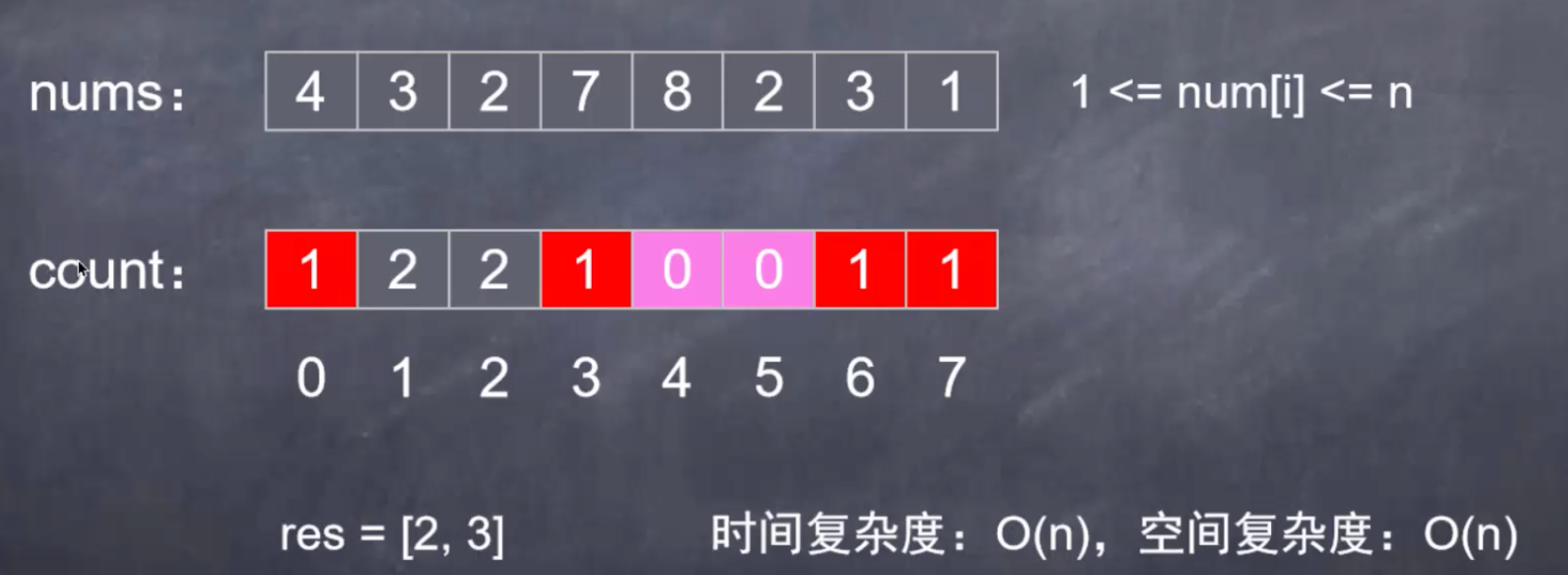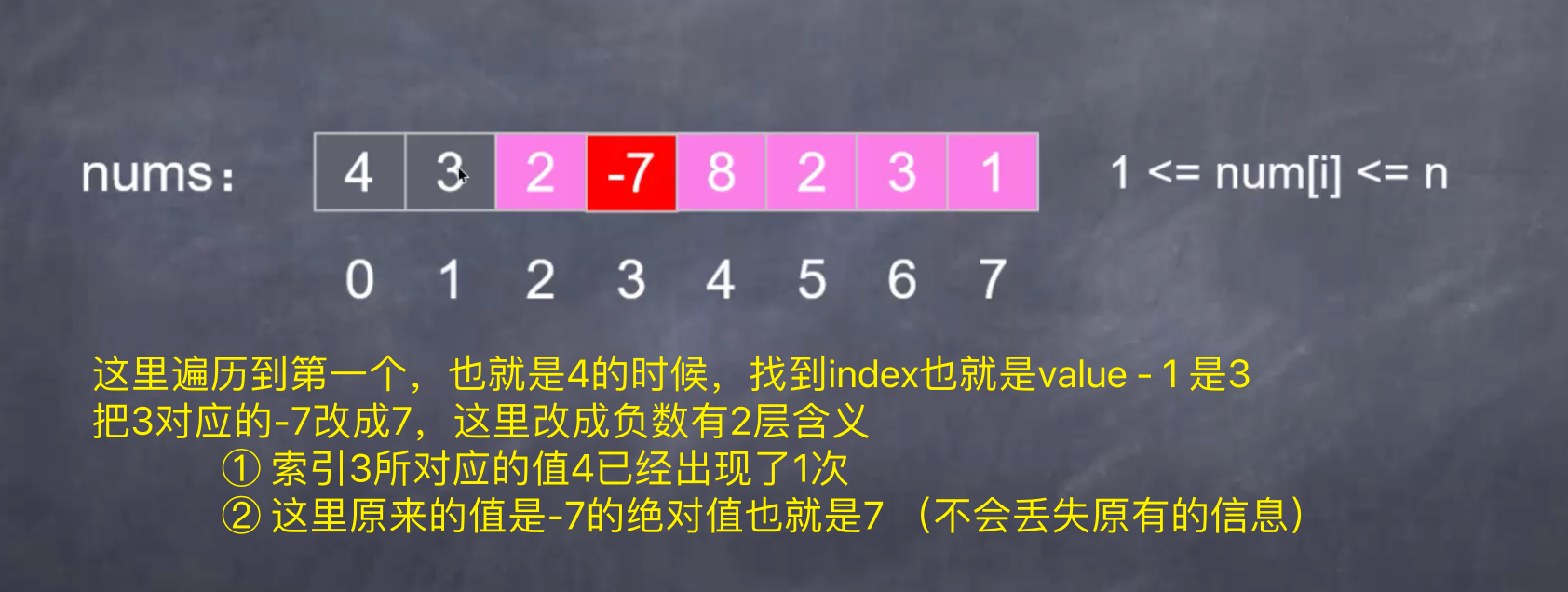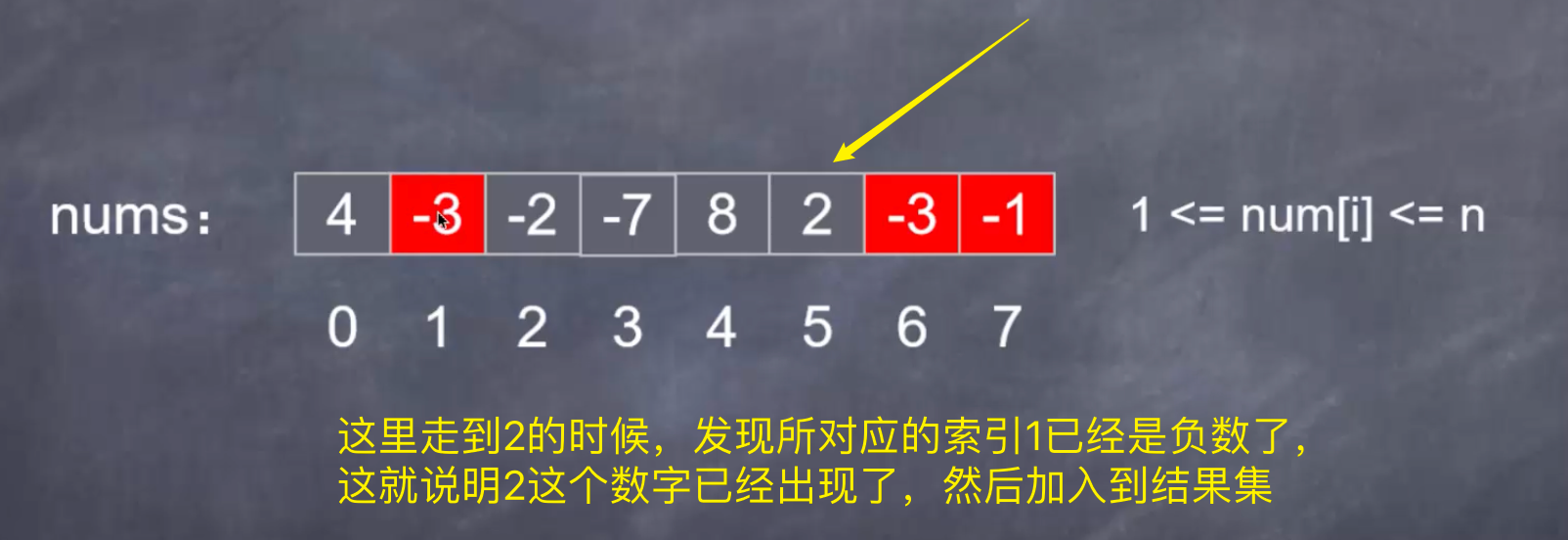res = [2, 3] 这样的空间复杂度也就是O(1)

#### 代码实现

Java&JavaScript&Python3

```class Solution {
public List<Integer> findDuplicates(int[] nums) {
List<Integer> res = new ArrayList<>(); // 结果集不算额外空间
for (int i = 0; i < nums.length; i++) {
// 这里必须取绝对值，有可能索引为负数
int index = Math.abs(nums[i]) - 1;
// 说明已经出现过了1次
if (nums[index] < 0) {
// 这里也必须取绝对值，不然索引是负数
// 原本的值添加到结果集
} else {
// 说明没有出现过，反转为负数
// 需要把索引对应的值翻转
nums[index] = -nums[index];
}
}
return res;
}
}
```
```/**
* @param {number[]} nums
* @return {number[]}
*/
var findDuplicates = function(nums) {
const res = [];
for(let i = 0; i < nums.length; i++) {
const index = Math.abs(nums[i]) - 1
if (nums[index] < 0) {
res.push(Math.abs(nums[i]));
} else {
nums[index] = -nums[index];
}
}
return res;
};
```
```class Solution:
def findDuplicates(self, nums: List[int]) -> List[int]:
res = []
for x in nums:
index = abs(x) - 1
if nums[index] < 0:
res.append(abs(x))
else:
nums[index] = -nums[index]
return res
```

### 解法2 还可以n倍

#### 思路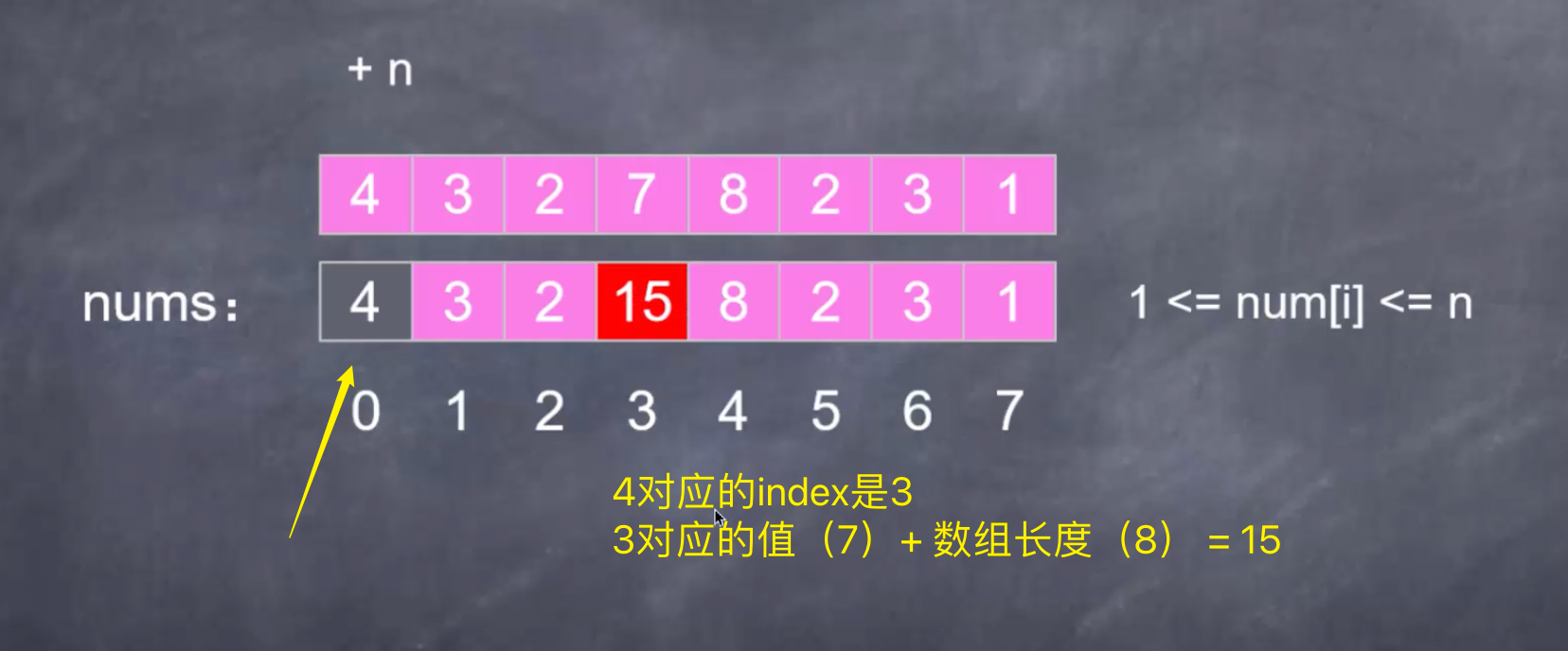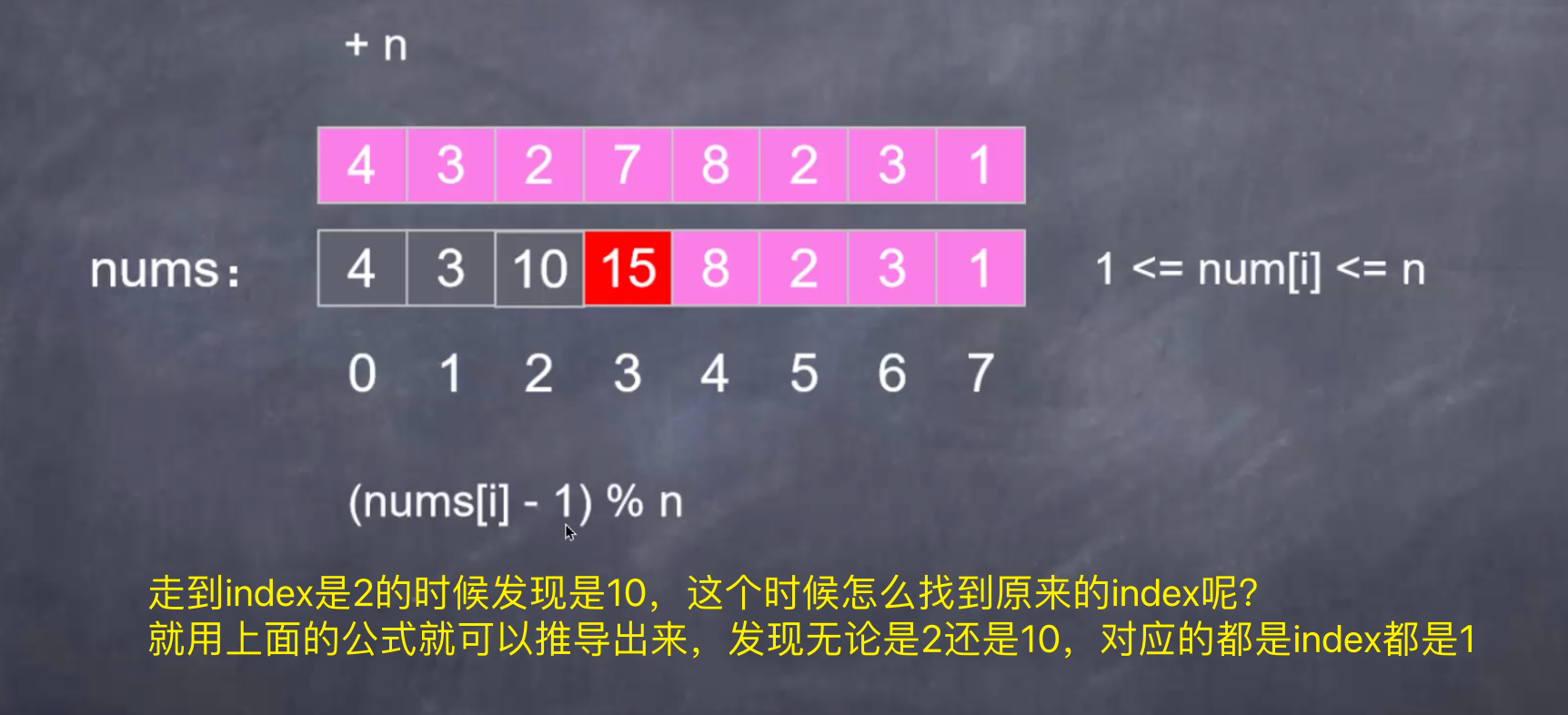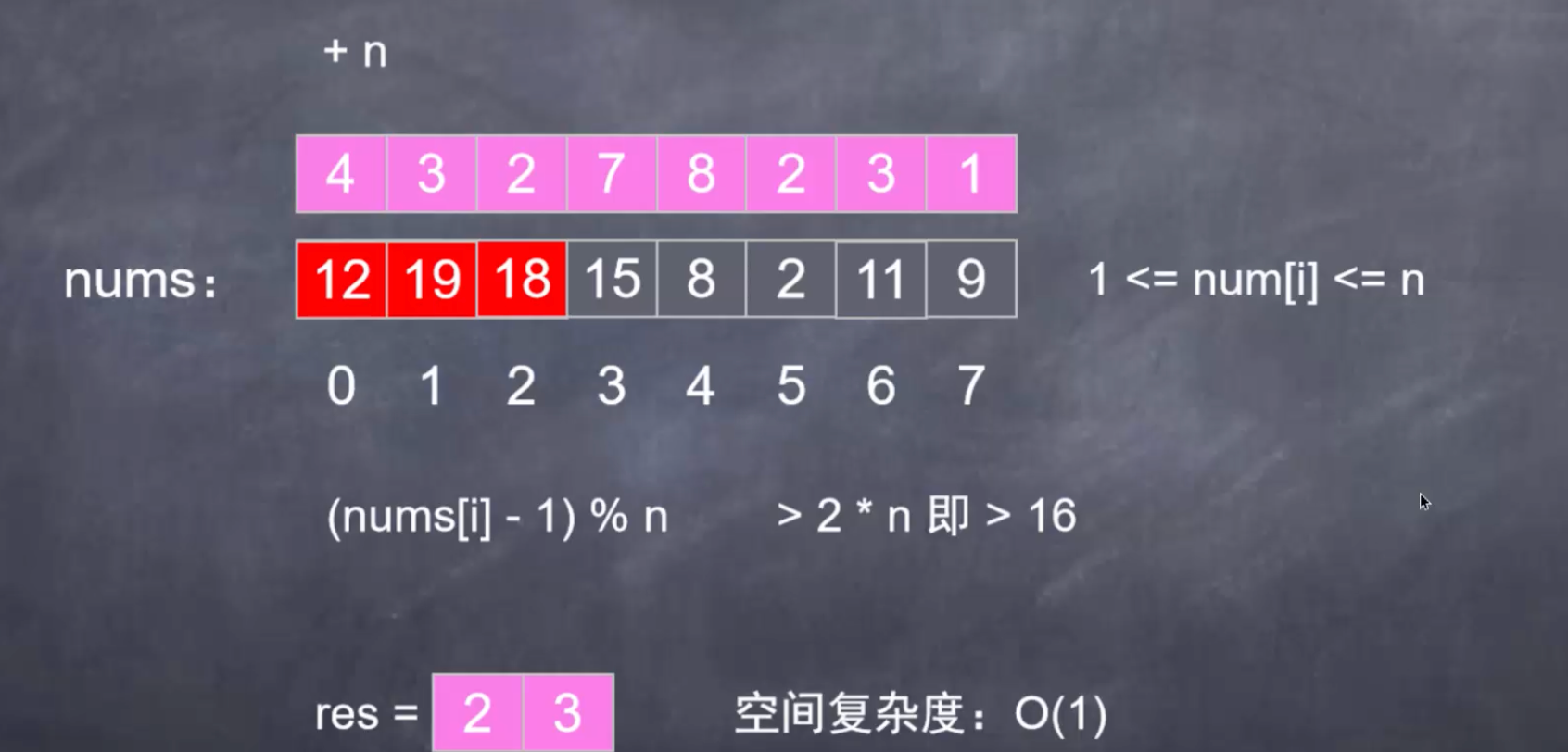• 遇到了 + n（长度）
• 最后判断是否重复就是2n

#### 代码实现

```class Solution {
public List<Integer> findDuplicates(int[] nums) {
int len = nums.length;
// 第一次遍历 重复的加倍
for (int i = 0; i < len; i++) {
// 由于这里可能是已经加倍过的，为了找到原来的index 需要这样计算
// 注意这里的值要-1，因为初始化的时候index比实际的值要-1，取模当然也要-1
int index = (nums[i] - 1) % len;
nums[index] += len;
}
// 第二次遍历 找到结果
List<Integer> res = new ArrayList<>();
// 这个时候的获得数组，index是值-1，value是加倍or不加倍，判断用的
for (int i = 0; i < len; i++) {
// 高于总长度2倍的，说明加了2次，也就是重复了2次
// 加入结果集的是值，而不是索引
// ∵ 但这个时候的索引其实就是值↓
// ∴ 索引+1=值
if (nums[i] > 2 * len) res.add(i + 1);
}
return res;
}
}
```